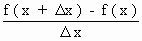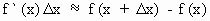Home MonkeyNotes Printable Notes Digital Library Study Guides Study Smart Parents Tips College Planning Test Prep Fun Zone Help / FAQ How to Cite New Title Request

5.15 Differentials : Approximation and Errors

Recall the definition of the derivative of a function y = f (x)This represents the slope of tangent to the curve y = f (x) at some point (x, f (x))

Now if Dx is very small (Dx ¹ 0), then the slope of tangent is approximately the same as the slope of the secant through (x, f (x))

i.e.\Now the differential of the independent variable x is written as dx and it is the same as Dx

\ dx = Dx , Dx ¹ 0

Hence, f  (x) . dx » f (x + Dx) - f (x) ... (1)

Similarly dy = Dy for the variable y, Dy ¹ 0.

we also have that Dy = f (x + Dx) - f (x)

\ dy = f (x + Dx) - f(x) ...(2)

Therefore from relations (1) and (2) we have dy = f  (x) dx = Dy

Thus, when the change in x (Dx = dx) is relatively very small, then the differential of y (dy) is approximately equal to the exact change in y (Dy) i.e. The smaller the change in x, the closer dy to Dy. This fact enables us to approximate the functional value close to f (x)Your browser does not support the IFRAME tag.

Example 47

Find dy if y = x2 + 7x + 2

Solution : Let y = f (x) = x2 + 7x + 2

\ f  (x) = 2x + 7

then dy = f  (x) dx

\ dy = ( 2x + 7 ) dx

Example 48

Find dy if y = cos x + sin 2x

Solution : Let y = f (x) = cos x + sin 2x

then dy = f  (x) dx

i.e. dy = ( 2 cos 2x - sin x ) dx

Approximations

Example 49

Find correct to 4 decimal places, the approximate value of 2x3 + 7x + 1 at x = 2.001

Solution : Let f (x) = 2x3 + 7x + 1 then f  (x) = 6x2 + 7

Then convenient value of x for f (x) is x = 2 which is relatively close to 2.001. \ f  (2) = 31

Also, x increases from x = 2 to x = 2.001

then we have Dx = 0.001

Now dy = f ` (x) . dx

\ dy = 31 . (0.001)

Now f (2) = 31

\ The approximate value of f (x) = 31 + 0.031 = 31.031

Index

5.1 Tangent And Normal Lines
5.2 Angle Between Two Curves
5.3 Interpretation Of The Sign Of The Derivative
5.4 Locality Increasing Or Decreasing Functions 5.5 Critical Points
5.6 Turning Points
5.7 Extreme Value Theorem
5.8 The Mean-value Theorem
5.9 First Derivative Test For Local Extrema
5.10 Second Derivative Test For Local Extrema
5.11 Stationary Points
5.12 Concavity And Points Of Inflection 5.13 Rate Measure (distance, Velocity And Acceleration)
5.14 Related Rates
5.15 Differentials : Errors And Approximation